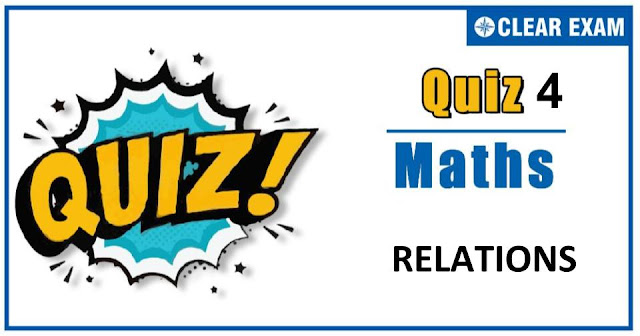## MATHEMATICS RELATIONS QUIZ-4Dear Readers,As per analysis for previous years, it has been observed that students preparing for JEE MAINS find Mathematics out of all the sections to be complex to handle and the majority of them are not able to comprehend the reason behind it. This problem arises especially because these aspirants appearing for the examination are more inclined to have a keen interest in Mathematics due to their ENGINEERING background. Furthermore, sections such as Mathematics are dominantly based on theories, laws, numerical in comparison to a section of Engineering which is more of fact-based, Physics, and includes substantial explanations. By using the table given below, you easily and directly access to the topics and respective links of MCQs. Moreover, to make learning smooth and efficient, all the questions come with their supportive solutions to make utilization of time even more productive. Students will be covered for all their studies as the topics are available from basics to even the most advanced.

Q1.
•  (0,∞)
•  (-∞,∞)
•  [0,1]
•  [-1/3,1]
Solution
Here, we have to find the range of the function which [-1/3 ,1]

Q2. f(x)=√(sin-1((log2⁡x)) ) exists for
•  x∈(1,2)
•  x∈[1,2]
•  x∈[2,∞)
•  x∈(0,∞)
Solution
We observe that √(sin-1⁡(log2⁡x ) ) exists for sin-1⁡((log2⁡x))≥0
i.e. for 0≤ log2⁡(x≤1⇒20 )≤x≤2⇒1≤x≤2

Q4. Let the function f(x)=x2+x+sin⁡(x-cos⁡(x+log⁡(1+|x| ) ) ) be defined on the interval [0,1]. The odd extension of f(x) to the interval [-1,1] is
•  x2+x+sin⁡(x+cos⁡(x-log⁡(1+|x| ) ) )
•  -x2+x+sin⁡(x+cos⁡(x-log⁡(1+|x| ) ) )
•  -x2+x+sin⁡(x-cos⁡(x+log⁡(1+|x| ) ) )
•  None of these
Solution

Q7.
• R-{-π,π}
•  R-{n π│n∈Z}
•  R-{2n π│n∈z}
•  (-∞,∞)
Solution

Q9.If f(x)=a x+b and g(x)=c x+d, then f(g(x) )=g(f(x) )⇔
•  f(a)=g(c)
•  f(b)=g(b)
•  f(d)=g(b)
•  f(c)=g(a)
Solution
We have,
f(x)=ax+b,g(x)=c x+d
∴f(g(x) )=g(f(x) )
for all x ⇔f(c x+d)=g(a x+b)
for all x ⇔a(c x+d)+b=c(a x+b)+d
for all x ⇔ad+b=c b+d [Putting x=0 on both sides] ⇔f(d)=g(b)

Q10.
•  (-3,-1)∪(1,∞)
•  [-3,-1)∪[1,∞)
•  (-3,-2)∪(-2,-1)∪(1,∞)
•  −3,−2∪−2,−1∪[1,∞)
Solution#### Written by: AUTHORNAME

AUTHORDESCRIPTION## Want to know more

Please fill in the details below:

## Latest NEET Articles\$type=three\$c=3\$author=hide\$comment=hide\$rm=hide\$date=hide\$snippet=hide

Name

ltr
item
BEST NEET COACHING CENTER | BEST IIT JEE COACHING INSTITUTE | BEST NEET & IIT JEE COACHING: RELATIONS-QUIZ-4
RELATIONS-QUIZ-4
https://1.bp.blogspot.com/-iD2fIjqnRYU/X37e67ug7DI/AAAAAAAABO8/1f66TItCzb4lj7duAH25lEujSnQn_T7AgCLcBGAsYHQ/w640-h336/Quiz%2BImage%2BTemplate%2B%252811%2529.jpg
https://1.bp.blogspot.com/-iD2fIjqnRYU/X37e67ug7DI/AAAAAAAABO8/1f66TItCzb4lj7duAH25lEujSnQn_T7AgCLcBGAsYHQ/s72-w640-c-h336/Quiz%2BImage%2BTemplate%2B%252811%2529.jpg
BEST NEET COACHING CENTER | BEST IIT JEE COACHING INSTITUTE | BEST NEET & IIT JEE COACHING
https://www.cleariitmedical.com/2020/10/RELATIONS-QUIZ-4.html
https://www.cleariitmedical.com/
https://www.cleariitmedical.com/
https://www.cleariitmedical.com/2020/10/RELATIONS-QUIZ-4.html
true
7783647550433378923
UTF-8

STAY CONNECTED